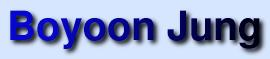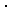Boyoon Jung's Library Manual

bj::KDClusterSimple Class Reference [Machine Learning Techniques]

A fast data clustering algorithm using a kernel density tree. More...

#include <kd_cluster.h>

List of all members.

Public Member Functions

KDClusterSimple (int dimension, double threshold, int max_clusters=10)
A constructor.
~KDClusterSimple (void)
A destructor.
virtual int cluster (KDTree *tree)
Cluster data points using multiple gaussians.
int numClusters (void)
Return the number clusters.
double * center (int c)
Return the center of a specific cluster.
double * boundary (int c)
Return the rectangle boundary of a specific cluster.
double densityThreshold (void)
Return the density threshold.
void setDensityThreshold (double th)
Set a new density threshold.

Protected Member Functions

double distance (gsl_vector *x, gsl_vector *y)
Compute the manhattan distance between two nodes.
void select_candidates (kd_node *tree, int npoints, kd_node **candidates, int &size)
Select candidate nodes from a kd-tree.

Protected Attributes

int nclusters
Number of clusters.
double ** ctr
Centers of clusters.
double ** rbs
Rectangle boundaries of clusters.
int dimension
The dimension of input points.
int max_clusters
Maximum number of clusters.
double threshold
Density threshold.

Detailed Description

A fast data clustering algorithm using a kernel density tree.

KDClusterSimple class implements a fast data clustering algorithm using a KD-tree. The clustering result is multiple, independent rectangles.

This class is mainly for clustering particles generated by AdaptiveParticleFilter class. It takes a kd-tree as an input instead of building one internally because AdaptiveParticleFilter class build a tree anyway to compute a proper number of particles.

Constructor & Destructor Documentation

 bj::KDClusterSimple::KDClusterSimple ( int dimension, double threshold, int max_clusters = 10 )

A constructor.

Parameters:
 dimension The dimension of data points. threshold The density threshold. max_clusters The maximum number of clusters.

 bj::KDClusterSimple::~KDClusterSimple ( void )
 A destructor.

Member Function Documentation

 double* bj::KDClusterSimple::boundary ( int c ) [inline]
 Return the rectangle boundary of a specific cluster. Returns:A double-type array whose length is 4:  left top right bottom

 double* bj::KDClusterSimple::center ( int c ) [inline]
 Return the center of a specific cluster. Returns:A double-type array whose length is 2:  x position y position

 virtual int bj::KDClusterSimple::cluster ( KDTree * tree ) [virtual]
 Cluster data points using multiple gaussians. Returns:The number of clusters.

 double bj::KDClusterSimple::densityThreshold ( void ) [inline]
 Return the density threshold.

 double bj::KDClusterSimple::distance ( gsl_vector * x, gsl_vector * y ) [inline, protected]
 Compute the manhattan distance between two nodes.

 int bj::KDClusterSimple::numClusters ( void ) [inline]
 Return the number clusters.

 void bj::KDClusterSimple::select_candidates ( kd_node * tree, int npoints, kd_node ** candidates, int & size ) [protected]
 Select candidate nodes from a kd-tree.

 void bj::KDClusterSimple::setDensityThreshold ( double th ) [inline]
 Set a new density threshold.

Member Data Documentation

 double** bj::KDClusterSimple::ctr [protected]
 Centers of clusters.

 int bj::KDClusterSimple::dimension [protected]
 The dimension of input points.

 int bj::KDClusterSimple::max_clusters [protected]
 Maximum number of clusters.

 int bj::KDClusterSimple::nclusters [protected]
 Number of clusters.

 double** bj::KDClusterSimple::rbs [protected]
 Rectangle boundaries of clusters.

 double bj::KDClusterSimple::threshold [protected]
 Density threshold.

The documentation for this class was generated from the following file:
ResearchProjectsPublications

ToolsBjLib ManualPlayer/StagePyPlayerMicroStrain IMUGPS/DGPSParticle Filter

PersonalWelcomeCurriculum VitaeResumeContactUSCUSC CS DeptCRESRobotics LabRESL